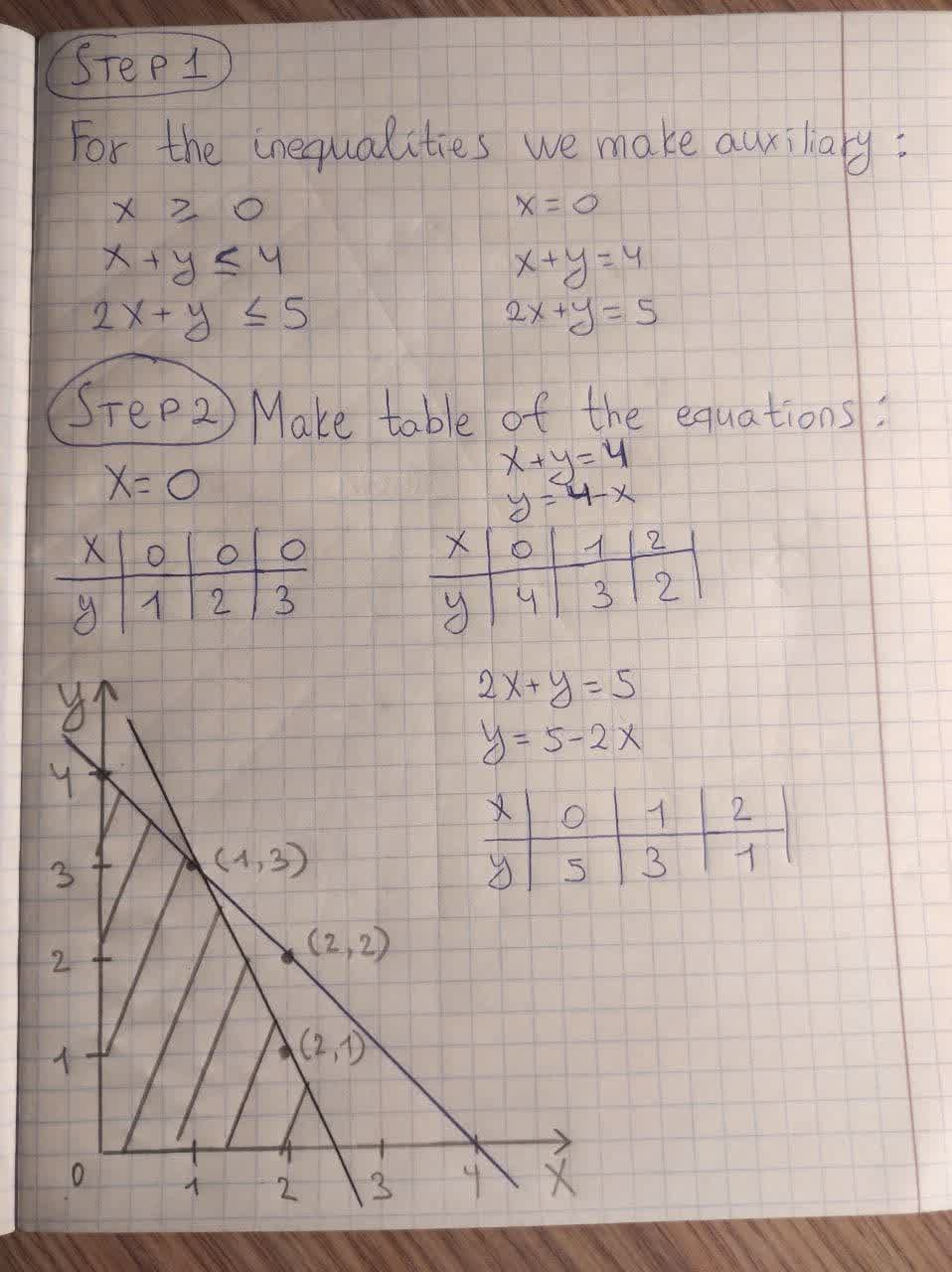# Graph the solution set of each system if inequalities. X is greater than or = 0 X+Y is less than or equal to 4 2x+y is less than or equal to 5alesterp 2021-01-31 Answered
Graph the solution set of each system if inequalities.
X is greater than or = 0
X+Y is less than or equal to 4
2x+y is less than or equal to 5
You can still ask an expert for help

## Want to know more about Inequalities systems and graphs?

• Questions are typically answered in as fast as 30 minutes

Solve your problem for the price of one coffee

• Math expert for every subject
• Pay only if we can solve itBella

Step 1
For the inequalities we make auxiliary equations.
x=0 ,x+y=4, 2x+y=5
$x\ge 0$
$x+y\le 4$
$2x+y\le 5$
x=0
x+y=4
2x+y=5
Step 2
Then we make table for the equations and graph:Step 4
Then shade the regions according to the inequalities.
For $x\ge 0$, shade the right side of x=0
For $x+y\le 4$, shadetowards (0,0) because x=0, y=0 satisfies the inequality.
For $2x+y\le 5$, shade towards (0,0) because x=0, y=0 satisfies the inequality.
So the common region is the shaded region in black.